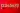# Learn Prime Numbers 1 to 100In mathematics, any number, specifically whole numbers which are divisible by the number 1 and itself is known as a prime number.

The prime numbers from 1 to 100 in total are 25. We can find a prime number with the help of a process known as prime factorization or integer factorization.

According to experts at a leading international school Singapore, children are taught prime numbers from a very young age. If you send your child to a good educational institution, they will be able to learn about the magic of prime numbers. When they advance in their life, they will be able to make use of the same to improve their professional and personal learning and growth.

A prime number will always consist of two factors; the number 1 and itself. For example; let us take a prime number ‘3’, if we find the factors of 3, we get ‘1’ and ‘3’, thus a prime number always has two factors. In this article, we will try to cover various topics related to prime numbers such as the properties of these numbers, facts related to them, and do a detailed analysis about them.

## Prime NumberAs mentioned above, any number which is divisible by the number 1 and itself is known as a prime number. For example ‘5’, the number five is only divisible by 1 and itself, thus it is considered as a prime number. One unique fact about prime numbers is that it consists of only two factors.

You should also remember that the numbers, zero and one are not prime numbers as they have only one factor or no factor. These numbers ( 0 and 1) are neither considered prime numbers nor composite numbers. Some examples of prime numbers are as follows, 5, 7, 71, 73, 89, 97, etc.

## Some important Properties Related to Prime NumbersThere are numerous properties of a prime number, in the next paragraph, we will try to see some of the significant properties of a prime number. Some of the properties of a prime number are as follow;

###### 4 Ways To Improve Your Medical School Application

1. A prime number will always be divisible by itself and the number 1. Thus, it will consist of two factors only. For example, ‘5’, which is only divisible by 1 and itself.
2. The numbers which are prime must always be positive and greater than 1. For example, 3, 5, 7, 23, 29, etc.
3. A non-zero whole number is always considered a prime number.
4. The only way of finding prime numbers is by the process of prime factorization or integer factorization.

## Some Significant Facts About Prime NumbersIn the paragraph mentioned above, we came to know about various significant properties of a prime number. The following points mentioned below analyses the important and unique facts about prime numbers;

1. Every even number is not a prime number except 2. In the number line, only 2 is a number that is considered as a prime number as it is divisible by and itself. Except for the number two, every even number has more than two factors. For example, let us take an even number ‘4’, this number has more than two factors; ‘1’, ‘2’, and 4. Thus, this even number is not considered a prime number.
2. You will find that, not a single prime number that is greater than 5 ending with a 5. No number above 5 which ends with the digit 5 can be divisible by 5, thus it cannot be considered as a prime number.
3. The numbers ‘0’ and ‘1’ are not an example of prime numbers, as these numbers consist of only one factor or no factor. The numbers ‘1’ and ‘0’ are also not considered composite numbers as they are not divisible by numbers other than that of 1. We all know, any integer can be divided by a number other than 1, and the number itself is defined as a composite number. Thus ‘0’ and ‘1’ are not composite numbers.

If you want to learn about prime numbers in a detailed manner, in an interesting manner, you may visit the website of Cuemath website.